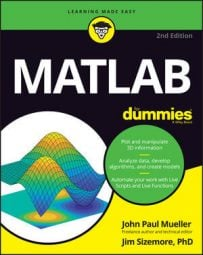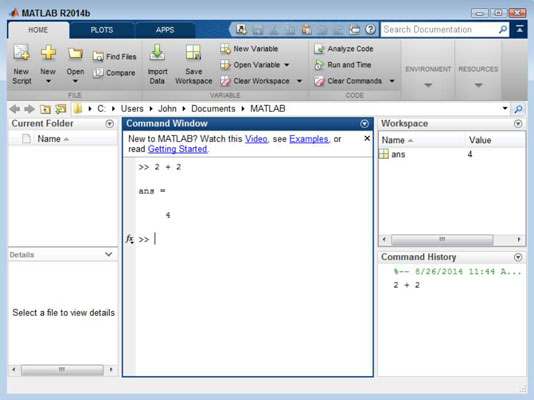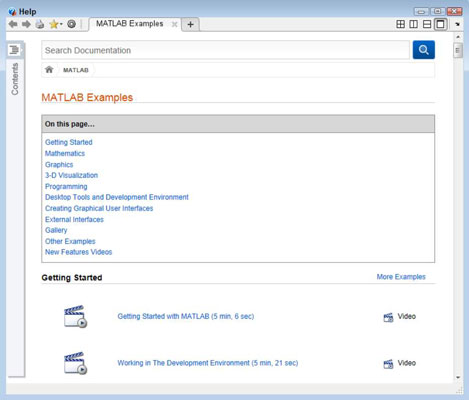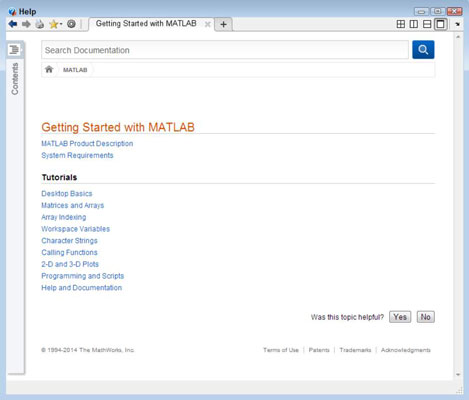##### MATLAB For DummiesThe MATLAB Command window is where you perform most of your experimentation. While you perform really simple tasks using the Command window, it can do quite a lot for you. Here are some of the ways in which you can use the Command window to learn more about MATLAB.

## Typing a really simple command

You can type any formula or command desired in the Command window and see a result. Of course, it pays to start with something really simple so that you can get the feel of how this window works. Type 2 + 2 and press Enter in the Command window.

Notice that it isn’t just the Command window that is affected when you type a formula or command. Other windows are changed as well. Here are the all windows affected:• Command window: Receives the output of the formula 2 + 2, which is ans = 4. MATLAB assigns the output of the formula to a variable named ans. Variables are boxes (pieces of memory) in which you can place data. In this case, the box contains the number 4.

• Workspace window: Contains any variables generated as the result of working in the Command window. In this case, the Workspace window contains a variable named ans that holds a value of 4.

Notice that the variable can’t contain any other value than 4 because the Min column also contains 4, as does the Max column. When a variable can contain a range of values, the minimum value that it can contain appears in the Min column and the maximum value that it can contain appears in the Max column. The Value column always holds the current value of the variable.

• Command History window: Displays the series of formulas or commands that you type, along with the date and time you typed them. You can replay a formula or command in this window. Just select the formula or command that you want to use from the list to replay it.

At the top of the Command window, notice three links that you can use to quickly get additional help in using MATLAB. Each link helps you in a different way, so you can use the links as needed to meet your specific needs. Here is an overview of what each link provides:

• Watch This Video: Opens a tutorial in your browser. The video provides a brief introduction to MATLAB. Simply watch it for a visual presentation of how to work with MATLAB.

• See Examples: Displays a Help dialog box that contains an assortment of examples that you can try. The examples take a number of forms:

• Video: Displays a guided presentation of how to perform a task that opens in your browser. The length of time of each video is listed next to its title.

• Script: Opens the Help dialog box to a new location that contains an example script that demonstrates some MATLAB feature and an explanation of how the script works. You can open the script and try it yourself. Making changes to the script is often helpful to see how the change affects script operation.

• App: Starts a fully functional app that you can use to see how MATLAB works and what you can expect to do with it.• Read Getting Started: Displays a Help dialog box that contains additional information about MATLAB, such as the system requirements. You also gain access to a number of tutorials.